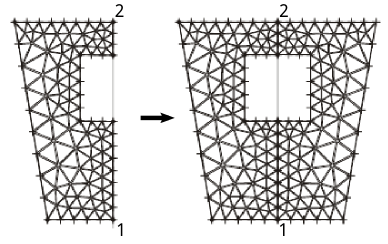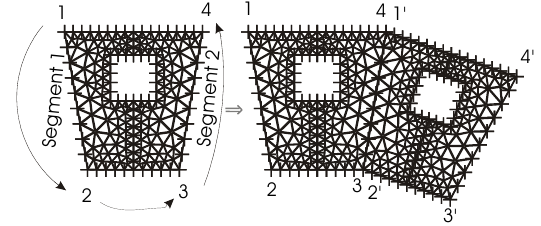# Node chains (Knotenketten)¶

Command: ndt(dist)

Sets the reference node-spacing. If nc_line or nc_circle specifies the number of nodes as 0, the number of nodes will be determined by the reference node spacing.

Parameter

dist Distance in global units

Command: nc_line(x1, y1, x2, y2, nodes)

Create a node-chain

Parameter
x1,y1 Start-node coordinates
x2,y2 End-node coordinates
nodes No. of nodes

Command: nc_line_cont(x, y, nodes)

Continue a node-chain

Parameter
x,y End-node coordinates
nodes No. of nodes

Function: nodesgen = nc.circle(x1, y1, x2, y2, nodes)

Create a node-chain on a circular segment defined by the points p1=(x1,y1) and p2=(x2,y2) defined in Cartesian coordinates. The circular segment is created starting at p1 in a mathematically positive direction around the origin of the reference system. A full circle is created if p1 = p2, or if p2 is not specified as an argument. If nodes=0, the number of nodes is set according to the reference node-spacing; (see ndt command).

Parameter
x1,y1 Start-point coordinates
x2,y2 End-point coordinates (following the mathematically positive direction)
nodes No. of nodes
Return value

nodesgen Number of nodes created (in case nodes = 0)

Examples:

Arc between points p1=(x1,y1) and p2=(x2,y2) with 20 nodes

```nc_circle(x1,y1,x2,y2,20)
```

Arc between points p1=(x1,y1) and p2=(x2,y2) with the node spacing defined by ndt. The number of nodes generated is returned in N.

```N = nc_circle(x1,y1,x2,y2,0)
```

Full circle with 100 nodes

```nc_circle(x1,y1,100)
```

Function: nodesgen = nc_circle_m(x1, y1, x2, y2, xm, ym, rm, nodes)

Create a node-chain on a circular segment defined by the points p1=(x1,y1) and p2=(x2,y2) around the centre pm=(xm,ym) defined in Cartesian coordinates with a radius rm. Some arguments are optional. The circular segment is created starting at p1 in a mathematically positive direction around the centre. A full circle is created if p1 = p2, or if p2 is not specified as an argument. If nodes=0, the number of nodes is set according to the reference node-spacing; (see ndt command).

Parameter
x1,y1 Start-point coordinates
x2,y2 End-point coordinates (following the mathematically positivedirection)
xm,ym Middle-point
nodes No. of nodes
Rückgabewerte

nodesgen Number of nodes created (in case nodes = 0)

Beispiele:

Arc between points p1=(x1,y1) and p2=(x2,y2) with 20 nodes

```nc_circle_m(x1,y1,x2,y2,xm,ym,20)
```

Arc between points p1=(x1,y1) and p2=(x2,y2) with the node spacing defined by ndt. The number of nodes generated is returned in N.

```N = nc_circle_m(x1,y1,x2,y2,xm,ym,0)
```

Full circle with 100 nodes around the center pm=(xm,ym) starting with the point p1=(x1,y1).

```nc_circle_m(x1,y1,xm,ym,100)
```

Full circle with 100 nodes around the center pm=(xm,ym) starting with the point p1=(x1,y1)with the radius r.

```nc_circle_m(xm,ym,r,100)
```

Command: mirror_nodechains(x1, y1, x2, y2)

Mirroring nodechain structures including mesh. The points of the mirror axis are given in counter-clockwise direction to the corners of the area to be mirrored.Point 1 is the lower right corner and point 2 the upper right corner of the area in the example. If it should be mirrored on the left edge, then point 1 is the upper left corner and point 2 the lower left. Only the starting and end point of the node chains have to be on the mirroring axis.

Parameter
x1,y1 Point 1
x1,y2 Point 2 of the mirroring axis

Command: rotate_copy_nodechains(x1, y1, x2, y2, x3, y3, x4, y4, count)

Rotating and copying of the node chain structure including the mesh. The points 1 to 4 are to be given in counter clockwise direction to the area to be copied. Point 1 and 2 define the edge segment to be copied and points 3 and 4 define the borderline to be expanded.Parameter
x1,y1 Point 1
x2,y2 Point 2
x3,y3 Point 3
x4,y4 Point 4
count No. of copies

Command: translate_copy_nodechains(x1, y1, x2, y2, x3, y3, x4, y4, count)

Moving and copying of the node chain stuctures including the mesh. The points 1 to 4 are to be given in counter clockwise direction to the area to be copied. Die Punkte 1 bis 4 sind bezüglich der Ecken des zu vervielfältigenden Gebietes entgegen dem Uhrzeigersinn anzugeben. Point 1 and 2 define the edge segment to be copied and points 3 and 4 define the borderline to be expanded.

Parameter
x1,y1 Point 1
x2,y2 Point 2
x3,y3 Point 3
x4,y4 Point 4
count No. of copies

Command: del_nc_single(x1, y1)

Deleting of a node chain including its superelements.

Parameter

x1,y1 Point near the node chain to be deleted.